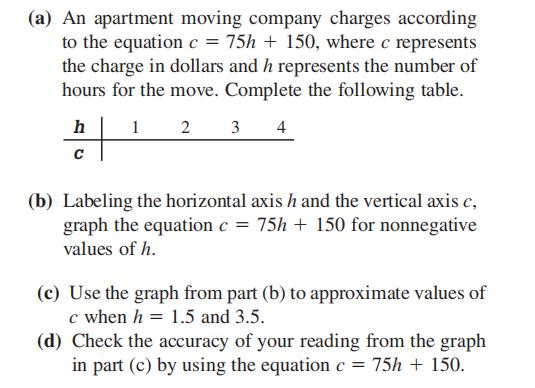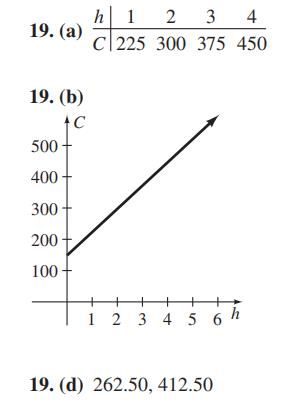### Still have math questions?

Algebra
Question(a) An apartment moving company charges according to the equation $$c = 75 h + 150$$ , where $$c$$ represents the charge in dollars and $$h$$ represents the number of hours for the move. Complete the following table.

(b) Labeling the horizontal axis $$h$$ and the vertical axis $$c$$ , graph the equation $$c = 75 h + 150$$ for nonnegative values of $$h$$ .

(c) Use the graph from part (b) to approximate values of

$$c$$ when $$h = 1.5$$ and $$3.5$$ .

(d) Check the accuracy of your reading from the graph in part (c) by using the equation $$c = 75 h + 150$$ .